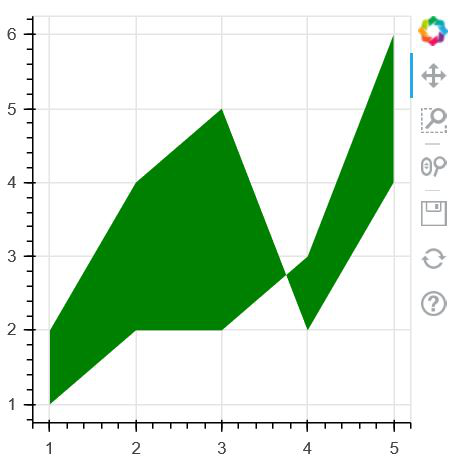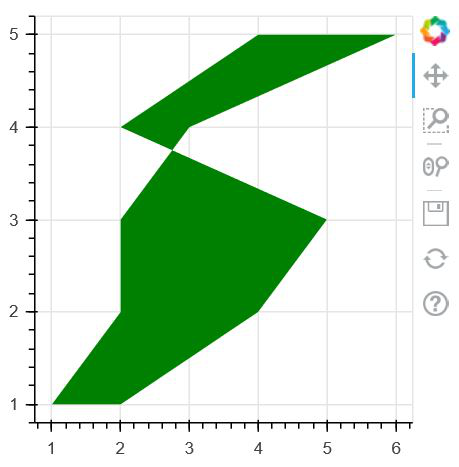Related Articles

# Make an area plot in Python using Bokeh

• Last Updated : 22 Jun, 2020

Bokeh is a Python interactive data visualization. Unlike Matplotlib and Seaborn, Bokeh renders its plots using HTML and JavaScript. It targets modern web browsers for presentation providing elegant, concise construction of novel graphics with high-performance interactivity.

## Plotting the Area Plots

Area plots are defined as the filled regions between two series that share a common areas. Bokeh Figure class has two methods which are given below:

• varea()
• harea()

1. varea() method:  varea() method is a vertical directed area which has one x coordinate array and two y coordinate arrays, y1 and y2, that will be filled between.

Syntax: varea(x, y1, y2, **kwargs)

Parameter:This method accept the following parameters that are described below:

• x: This parameter is the x-coordinates for the points of the area.
• y1: This parameter is the y-coordinates for the points of one side of the area.
• y2: This parameter is the y-coordinates for the points of other side of the area.

Example:

## Python3

 `# Implementation of bokeh function``   ` `import` `numpy as np ``from` `bokeh.plotting ``import` `figure, output_file, show``   ` `x ``=` `[``1``, ``2``, ``3``, ``4``, ``5``]``y1 ``=` `[``2``, ``4``, ``5``, ``2``, ``4``]``y2 ``=` `[``1``, ``2``, ``2``, ``3``, ``6``]``  ` `output_file(``"geeksforgeeks.html"``)``  ` `p ``=` `figure(plot_width``=``300``, plot_height``=``300``)``  ` `# area plot``p.varea(x``=``x, y1``=``y1, y2``=``y2,fill_color``=``"green"``)``  ` `show(p)`

Output:2. harea() method:  harea() method is a horizontal directed area which has one x coordinate array and two y coordinate arrays, y1 and y2, that will be filled between.

Syntax: harea(x1, x2, y, **kwargs)

Parameter:This method accept the following parameters that are described below:

• x1: This parameter is the x-coordinates for the points of one side of the area.
• x2: This parameter is the x-coordinates for the points of other side of the area.
• y: This parameter is the y-coordinates for the points of the area.

Example:

## Python3

 `# Implementation of bokeh function``   ` `import` `numpy as np ``from` `bokeh.plotting ``import` `figure, output_file, show``   ` `y ``=` `[``1``, ``2``, ``3``, ``4``, ``5``]``x1 ``=` `[``2``, ``4``, ``5``, ``2``, ``4``]``x2 ``=` `[``1``, ``2``, ``2``, ``3``, ``6``]``  ` `output_file(``"geeksforgeeks.html"``)``  ` `p ``=` `figure(plot_width``=``300``, plot_height``=``300``)``  ` `# area plot``p.harea(x1``=``x1, x2``=``x2, y``=``y,fill_color``=``"green"``)``  ` `show(p)`

Output:Attention geek! Strengthen your foundations with the Python Programming Foundation Course and learn the basics.

To begin with, your interview preparations Enhance your Data Structures concepts with the Python DS Course. And to begin with your Machine Learning Journey, join the Machine Learning – Basic Level Course

My Personal Notes arrow_drop_up